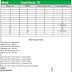Here is pairing scheme 2019 lastest for 10th class Lahore Board, Gujranwala Board, Multan Boaer and all Punjab boards. This pairing scheme is available to download in PDF from here. Zahid Notes provides all notes and guess in PDF for class 10.

Note:
These are old 2019 pairing schemes. The pairing schemes for 2020 have been posted in a different post the links are as follows:

## Class 10  pairing scheme 2019 PDF

Please see the new pairing scheme below.

## Math pairing scheme class 10 2019

MCQs (Total 15)2 MCQs from Chapter 2, 3, 5
1 MCQs from chapter 1, 4, 6, 7, 8, 9, 10, 11, 13
No MCQs from chapter 12

Short QuestionsQ.2. (6/9)
Chapter 1 = 2
Chapter 2 = 4
Chapter 3 = 3

Q.3. (6/9)
Chapter 4 = 2
Chapter 5 = 4
Chapter 6 = 3

Q.4. (6/9)
Chapter 7 = 2
Chapter 8 = 1
Chapter 9 = 1
Chapter 10 = 1
Chapter 11 = 1
Chapter 12 = 1
Chapter 13 = 2

Long Questions
Q.5. (Chapter 1+2)
Q.6. (Chapter 3+4)
Q.7. (Chapter 5+6)
Q.8. (Chapter 7+13)
Q.9. (Chapter 9 OR 12) (Theorem)

## Biology pairing scheme class 10 2019

MCQs (Total 12)2 MCQs from Chapter 12, 14, 15
1 MCQs from chapter 10, 11, 13, 16, 17, 18

#### Short Questions

Q.2. (5/8)
Chapter 10 = 2
Chapter 11 = 3
Chapter 12 = 3

Q.3. (5/8)
Chapter 13 = 2
Chapter 14 = 3
Chapter 15 = 3

Q.4. (5/8)
Chapter 16 = 2
Chapter 17 = 3
Chapter 18 = 3

Long Questions (2/3)
Q.5. (Chapter 11+12)
Q.6. (Chapter 13+14)
Q.7. (Chapter 16+17)
Note: No long question from chapters 10, 15, 18

New and Fresh:

## 10th class physics pairing scheme 2019

MCQs (Total 12)2 MCQs from Chapter 12, 14, 15
1 MCQs from chapter 10, 11, 13, 16, 17, 18

Short Questions
Same as biology pairing

Long Questions (2/3)
Same as Biology Pairing
Note: No long question from chapters 10, 15, 18

## 10th class Chemistry pairing

MCQs (Total 12)2 MCQs from Chapter 9, 10, 13, 15
1 MCQs from chapter 11, 12, 14, 16

Short QuestionsQ.2. (5/8)
Chapter 9 = 4
Chapter 10 = 4

Q.3. (5/8)
Chapter 11 = 3
Chapter 12 = 2
Chapter 13 = 3

Q.4. (5/8)
Chapter 14 = 3
Chapter 15 = 2
Chapter 16 = 3

Long Questions (2/3)
Q.5. (Chapter 9+10)
Q.6. (Chapter 12+13)
Q.7. (Chapter 15+16)
Note: No long question from chapters 11 and 14

## 10th class computer science pairing 2019

MCQs (Total 10)
2 MCQs from Chapter 1, 2, 4
1 MCQs from chapter 3, 5, 6, 7

Short Questions
Q.2. (4/6)
Chapter 1 = 4
Chapter 2 = 2

Q.3. (4/6)
Chapter 3 = 2
Chapter 4 = 2
Chapter 5 = 2

Q.4. (4/6)
Chapter 6 = 2
Chapter 7 = 4

Long Questions (2/3)
Q.5. (Chapter 1+2)
Q.6. (Chapter 4+5)
Q.7. (Chapter 6+7)
Note: No long question from chapters 11 and 14

### Pairing schemes:

Pairing scheme of 10th class 2019 NewReviewed by Saif Ullah ZahidonJanuary 16, 2019 Rating: 5